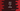# 3 different ways to copy a string in Java## How to copy a string in java :

String is immutable in Java. i.e. we can’t modify a string. Copying a string is required in many places and in this post we will learn how to copy a string in Java with example.

We will use == to compare two variables in this example. == is used to check if two variables are pointing to the same memory location or not. If it is not, both are different.

### Method 1: Using direct assignment:

If we directly assign one variable holding a string to another variable.

For example:

``````public class Main
{
public static void main(String[] args) {
String firstString = "Hello World";
String copyString = firstString;

System.out.println(firstString == copyString);
}
}``````

Here, we are not actually making a different copy of the string variable firstString. We are creating one different variable copyString and pointing it to the same memory location currently pointed by firstString.

Since string is immutable in Java, it can’t be changed. So, even if we change firstString to point to a different string, copyString will keep pointing to the current string and we got a backup for firstString.

If you run this program, it will print true because both are pointing to the same memory location.

### Method 2: Using StringBuffer :

Using the constructor of StringBuffer, we can copy the content of a string to a new StringBuffer object. Then, we can convert that StringBuffer to a String object using toString() method.

Let’s take a look at the below program:

``````public class Main
{
public static void main(String[] args) {
String firstString = "Hello World";
String copyString = new StringBuffer(firstString).toString();

System.out.println(firstString);
System.out.println(copyString);
System.out.println(firstString == copyString);
}
}``````

Here, we are creating the copyString variable by using a StringBuffer. If you run the above program, it will print the below output:

``````Hello World
Hello World
false``````

As you can see here, firstString and copyString holds the same value. But if we use ==, it prints false because both are pointing to different memory locations.

### Method 3: Using String.copyValueOf:

copyValueOf method is used to create a string from a character array. In our case, we can convert the string to a character array and pass that array to copyValueOf method.

Let’s take a look at the below program:

``````public class Main
{
public static void main(String[] args) {
String firstString = "Hello World";
String copyString = String.copyValueOf(firstString.toCharArray());

System.out.println(firstString);
System.out.println(copyString);
System.out.println(firstString == copyString);
}
}``````

This is similar to the above example. We are using copyValueOf to copy the content of a character array and creating one string. If you run the above program, it will print the below output:

``````Hello World
Hello World
false``````

It creates a different string object.